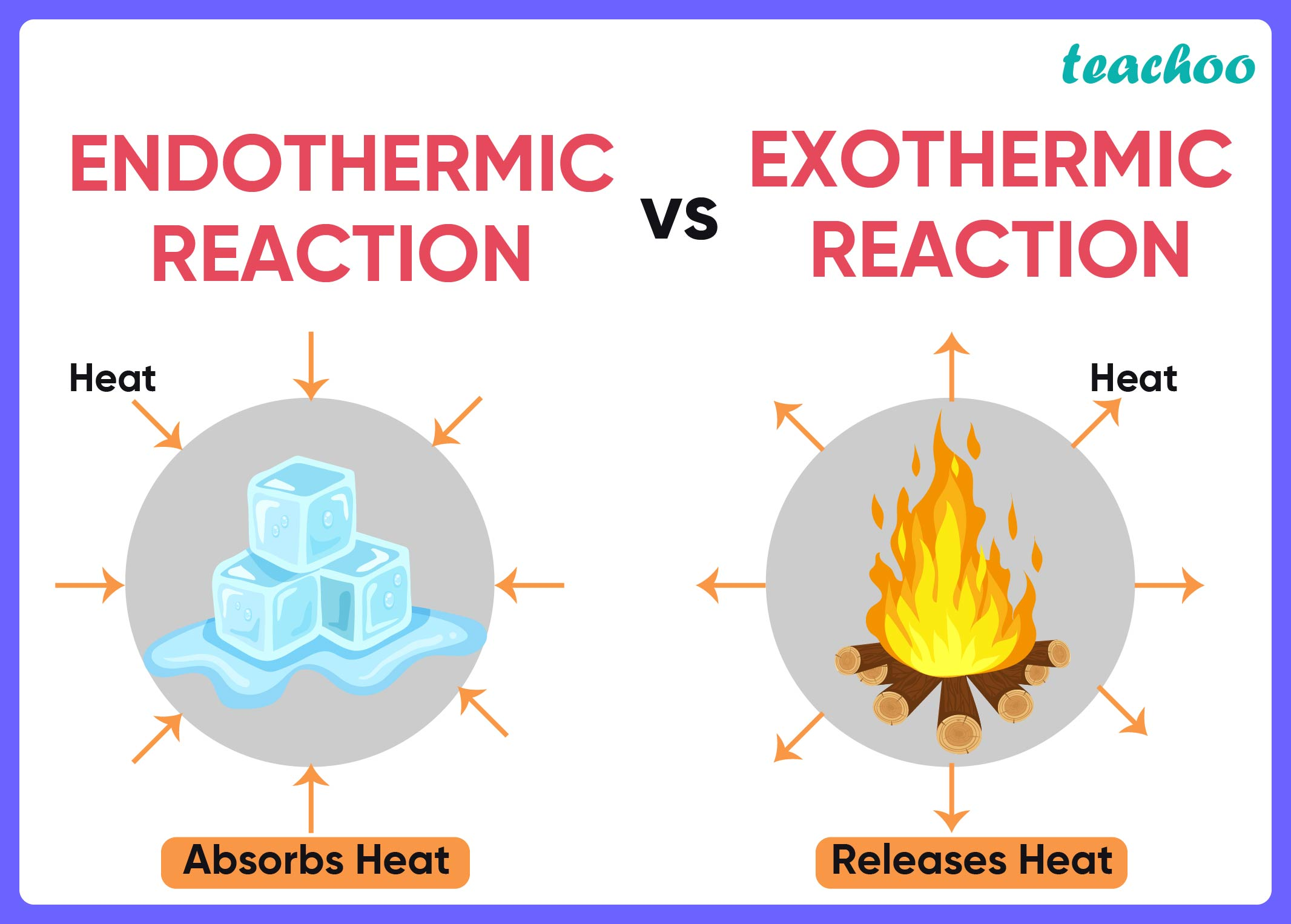MCQs from NCERT Exemplar

Class 10
Chapter 1 Class 10 - Chemical Reactions and Equations (Term 1)

## (D) (ii) and (iii)Here,

• Exothermic reactions are the ones where heat is evolved/given out .
• Endothermic reactions are the ones where heat is absorbed .

Now,

• Since the temperature of the solution in beakers A and B increased, an exothermic reaction took place in them.
• Also, because the temperature of the solution decreased in beaker C . So, endothermic reactio n took place

So, the correct option is (c) (i) and (iv)